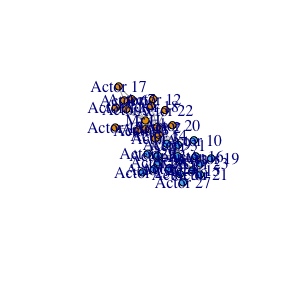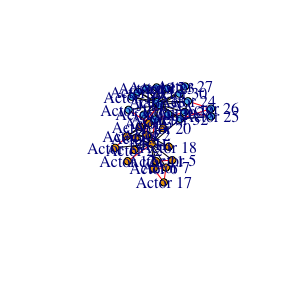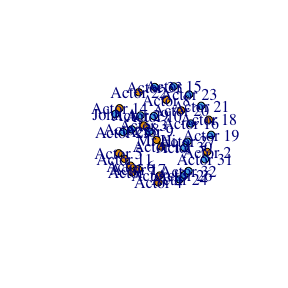# Finding Significant Links with gHypEGs

#### 20/4/2018

In this short tutorial we exploit the ghypernet package to detect significant links. We use the Karate Club as running example.

library(ghypernet)
library(igraph)
##
## Attaching package: 'igraph'
## The following objects are masked from 'package:stats':
##
##     decompose, spectrum
## The following object is masked from 'package:base':
##
##     union
data("adj_karate")
data("vertexlabels")
V(karate)$color <- vertexlabels ### Estimate the ensemble First we estimate a soft-configuration model from the data. The second model is a block model based on the two ‘factions’ of members of the club mod <- scm(adj_karate,directed = F, selfloops = F) blockModel <- bccm(adj = adj_karate, labels = vertexlabels, directed = F, selfloops = F, homophily = F) ## Block Model As the Karate Club consists of two factions, we can refine the model adding the block structure to it. Then we can filter out the links that are not significant according to this more complex model. What we expect in this case is that most of the within-group links are now not significant anymore, as they can be explained by the joint effect of configuration model and block model. Hence the links that remains after filtering are those that go beyond this joint effect. In particular, only few nodes of a group interact with the other group, i.e. the between-groups links are concentrated between few dyads. From the model instead we should expect most nodes of a group weakly interacting with the other group. Hence, we can expect that the significant links will be mainly those between groups. The results are in accordance with our intuition. signmat <- linkSignificance(adj_karate, blockModel, under=FALSE) # filter adjacency matrix adjfiltered <- adj_karate adjfiltered[signmat>(1/mod$m)] <- 0
adjfiltered[signmat<(1/mod$m) & adj_karate==0] <- 1 diag(adjfiltered) <- 0 adjcolor <- adj_karate adjcolor[adj_karate>0] <- 2 adjcolor[signmat<(1/mod$m)] <- 1
E(g)$color[E(g)$color==1] <- "red"
E(g)$color[E(g)$color==2] <- "black"

V(gfiltered)$color <- V(g)$color <- V(karate)\$color
plot(karate)plot(g)plot(gfiltered)